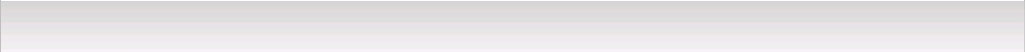﻿ Pan-Malaysia Global Taekwon-Do FederationSystem Of Rank | Patterns

TAEKWON-DO's SYSTEM OF RANK

In Taekwon-Do, character development, fortitude, tenacity, and technique are graded as well as individual capacity. The promotional scale is divided into nineteen ranks - 10 grades (Gups) and nine degrees (Dans.) The former begins with 10th grade (Gup) the lowest and ends at first grade. Degrees begin with the first degree (Dan) and end with the ultimate, ninth degree.

There is, of course, certain significance in the numbering system. With degrees, the number 9 is not only the highest one among one digit numbers but also is the number of 3 multiplied by 3. In the Orient, three is the most esteemed of all the numbers.

The Chinese character representing three is written: . The upper line symbolizes the heaven; the middle line, mortals; and the bottom line earth. It was believed that the individual who was successful in promoting his country, fellowmen and God, and able to reach an accord with all three would aspire to become King, which was written thusly: . The Chinese character for three and king are nearly synonymous.

When the number three is multiplied by itself, the equation is nine, the highest of the high; therefore ninth degree is the highest of the high-ranking belts. It is also interesting to note that when the number 9 is multiplied by any other single digit number and the resultant figures are added together, the answer always equals 9, i.e. 9x1=9; 9x2=18, 1+8=9 and so on up to 9x9=81, 8+1=9. Since this is the only single digit number having this property, it again points to the number 9 as being the most positive of figures. Taking the use of the number three one-step further, the degrees are further divided into three distinct classes.

First through third degree is considered the novice stages of black belt. Students are still merely beginners in comparison to the higher degrees. At fourth degree, the student crosses the threshold of puberty and enters the expert class. Seventh through ninth is composed of Taekwon-Do masters - the elite who fully understand all the particulars of Taekwon-Do, mental and physical.

There is perhaps one question that remains; why begin with the lowest of the two digit numbers, "10" why not begin with the lowest one digit number and proceed from first grade to ninth grade, and then begin again for degrees? Though it would certainly be more logical, the 10 to 1 and 1 to 10 numerical system in the Orient is ageless.

It would be impossible, if not even a bit impertinent, to attempt to change a practice that is even carried into children's games. Perhaps there was an initial logical reason for it: however, it seems to have been lost in antiquity. Anyhow, the number "10" is the lowest existing two-digit number: consequently, a beginner must start at this number rather than 11 or 12 which are numerically higher.

 System Of Rank | Patterns